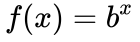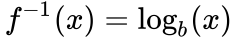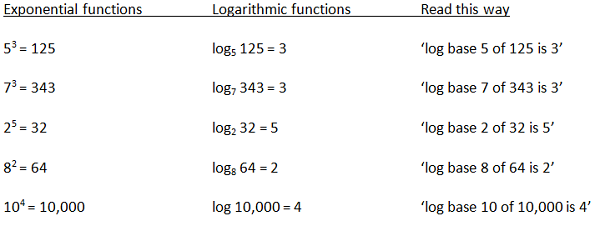# Logarithmic Function: Definition & Examples

An error occurred trying to load this video.

Try refreshing the page, or contact customer support.

Coming up next: What is an Inequality?

### You're on a roll. Keep up the good work!

Replay
Your next lesson will play in 10 seconds
• 0:04 Logarithmic Functions:…
• 0:25 Exponents vs. Logarithms
• 4:59 Logarithms in the Real World
• 6:41 Natural Logs Used with Base E
• 7:55 Lesson Summary

Want to watch this again later?

Timeline
Autoplay
Autoplay
Speed

#### Recommended Lessons and Courses for You

Lesson Transcript
Instructor: Ellen Manchester
Exponential functions and logarithmic functions are closely tied. In fact, they are so closely tied we could say a logarithm is actually an exponent in disguise. A logarithm, or log, is just another way to write an exponential function in reverse.

## Logarithmic Functions: Scary or Easy?

Some of you may find the term logarithm or logarithmic function intimidating. Relax! In this lesson, we are going to demystify the term and show you how easy it is to work with logarithms. To keep things simple, we will use the term logs when referring to logarithms or a logarithmic function.

## Exponents vs. Logarithms

An exponent is just a way to show repeated multiplication. For instance, in 32 = 3*3 = 9, the 3 is called the base of the exponent and the superscripted 2 is called the exponent or power. An exponential function tells us how many times to multiply the base by itself. Here are some examples:

• 53 = 5*5*5 = 25*5 =125 means take the base 5 and multiply it by itself three times.
• For 25, we take the 2 and multiply it by itself five times, like this: 2*2*2*2*2 = 4*2*2*2 = 8*2*2 = 16*2 = 32. This yields a result of 32.

An exponential function is written this way, where b is the base and x is the exponent.A logarithmic or log function is the inverse of an exponential function. We can use a log function to find an exponent. Let's use this information to set up our log. The logarithmic function is written this way:Notice that the b is the same in both the exponential function and the log function and represents the base. Here is an example of using the same set of information and expressing it as a log and an exponent:

Exponential function form: 32 = 9

Logarithmic function form: log base 3 of 9 = 2

Stop and take a look at both forms. In exponential function form, we have 9 as the answer. In the log form, the 2 is the answer and represents the exponent. What did we say was a log? A log is an exponent or in another format: log = exponent. Let's try a few more.Notice on the last logarithm that we did not include the base 10. Base 10 is called the common log. This is one of the most often used logs and is the base on all calculators with a log button. If you see a log written without a base, this is base 10.

To make sure you understand how to go from logs to exponents and back, try these:

• Write as an exponent: log base 10 of 100 = 2
• Write as an exponent: log base 5 of 25 = 2
• Write as a log: 93 = 729
• Write as a log: 62 = 36
• Write as a log: 100= 1
• Write as an exponent: log base 3 of 1/27 = -3

• 102 = 100
• 52 = 25
• log base 9 of 729 = 3
• log base 6 of 36 = 2
• Log base 10 of 10 = 1
• 3-3 = 1/27

Did you stumble on the last one? Remember exponents can also be negatives. A negative exponent just means the reciprocal. So 3-3 = 1/33 = 1/27.

To unlock this lesson you must be a Study.com Member.

### Register to view this lesson

Are you a student or a teacher?

### Unlock Your Education

#### See for yourself why 30 million people use Study.com

##### Become a Study.com member and start learning now.
Back
What teachers are saying about Study.com

### Earning College Credit

Did you know… We have over 160 college courses that prepare you to earn credit by exam that is accepted by over 1,500 colleges and universities. You can test out of the first two years of college and save thousands off your degree. Anyone can earn credit-by-exam regardless of age or education level.